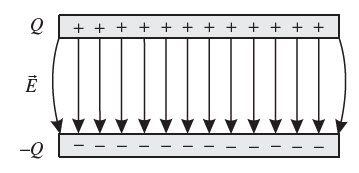# How voltage is related to Electrostatic Field

In electronics circuits, there is a close relationship between electric field and voltage. The electric field (E) and voltage (V) are interconnected and play crucial roles in the functioning of electronic devices. Understanding their relationship is fundamental to understanding how circuits work.

Electric field is a vector quantity that represents the force per unit charge experienced by a charged particle within an electric field. It is created by the presence of electric charges in a circuit. The force between two charges $$Q_1$$ and $$Q_2$$ separated by distance r is given by,

$$F = \frac{1}{4 \pi \epsilon_0}\frac{Q_1 Q_2}{r^2}$$ ----->(1)

where, $$\epsilon_0 = 8.85 \times 10^{-12} A s/V m$$ is the permittivity of free space.

The electric field strength due to charge $$Q_1$$ at the location of charge $$Q_2$$ is given by,

$$E_1 = \frac{F_2}{Q_2}$$   ---->(2)

From (1) and (2) we have,

$$E_1 = \frac{Q_1}{4 \pi \epsilon_0 r^2}$$   ---->(3)

The electric field lines show the direction and magnitude of the force that a positive charge would experience if placed at a particular point in the field.

Voltage, on the other hand, is the electric potential difference between two points in a circuit. It represents the work done per unit charge to move a charge from one point to another. Voltage is measured in volts (V) and is a scalar quantity.

Consider the charge $$Q_2$$ moves around within the electric field $$E_1$$ generated by the charge $$Q_1$$. The work done is moving the charge $$Q_2$$ around within the electric field $$E_1$$ from point $$r_a$$ and $$r_b$$ is,

$$W_{r_a r_b} = \int_{r_a}^{r_b} F_2 \,ds$$  --->(4)

using (2) we get,

$$W_{r_a r_b} = \int_{r_a}^{r_b} E_1 Q_2 \,ds$$

or, $$W_{r_a r_b} = Q_2 \int_{r_a}^{r_b} E_1 \,ds$$

or, $$W_{r_a r_b} = Q_2 V$$  --->(5)

where V is the voltageIn a circuit, voltage is directly related to the electric field. The electric field strength between two points in a circuit is directly proportional to the voltage difference between those points. Mathematically, from (5) the relationship between voltage and electric field can be expressed as:

E = V/d

where E is the electric field strength, V is the voltage, and d is the distance between the points. This equation indicates that the electric field strength is inversely proportional to the distance between the points.

This relationship implies that a higher voltage difference between two points in a circuit will result in a stronger electric field. Conversely, a smaller voltage difference will result in a weaker electric field. Therefore, voltage acts as a driving force for the electric field.

Furthermore, the electric field influences the movement of charges within a circuit. When a voltage is applied across a conductor, the electric field exerts a force on the free charges (electrons) within the material, causing them to move. This movement of charges constitutes an electric current. The current flow is proportional to the strength of the electric field, which, in turn, is related to the applied voltage.

In summary, the electric field and voltage in an electronic circuit are interconnected. Voltage creates an electric field, and the strength of the electric field is determined by the voltage difference between two points. The electric field, in turn, influences the movement of charges and the flow of current within the circuit. Understanding this relationship is crucial in analyzing and designing electronic circuits.